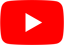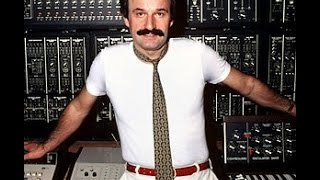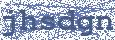E=mc2
Giorgio Moroder

## GIORGIO MORODER

E=mc2 Lyrics

ba da di di di
ba da da da da
bi di down down down

bri ba da da da da
ba ba ba ba ba ba
everybody di di di

Energy =
Mc squared
ba da da
Mc squared
ba da da

bi di di di
ba da da da
everybody dow dow dow
di di di di id
di da da da
everybody di di di

Energy =
MC squared
Energy =
MC squared
Energy =
MC squared
Energy =
MC squared

(Talking)

Energy =
MC squaredHottest Lyrics with Videos
e0864ccafa68fc77a78fafddc2414cff